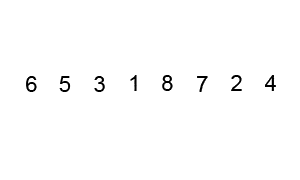# Bubble Sort In CBubble sort is a simple sorting algorithm that sorts the elements in ascending and descending order. It repeatedly compares the adjacent elements and swaps them if they are in the wrong order.

#### Example to understand Bubble Sort,

Suppose there is an array which contains ” 5 1 4 2 8″. If you want to sort this array in ascending order (lowest number to the greatest number), you need to take the following steps,

First Pass:

( 5 1 4 2 8 ) → ( 1 5 4 2 8 ), Here, the algorithm compares the first two elements and swaps since 5 > 1.
( 1 5 4 2 8 ) → ( 1 4 5 2 8 ), Swap since 5 > 4
( 1 4 5 2 8 ) → ( 1 4 2 5 8 ), Swap since 5 > 2
( 1 4 2 5 8 ) → ( 1 4 2 5 8 ), Now, since these elements are already in order (8 > 5), algorithm does not swap them.

Second Pass:

( 1 4 2 5 8 ) → ( 1 4 2 5 8 )
( 1 4 2 5 8 ) → ( 1 2 4 5 8 ), Swap since 4 > 2
( 1 2 4 5 8 ) → ( 1 2 4 5 8 )
( 1 2 4 5 8 ) → ( 1 2 4 5 8 )

Third Pass:

( 1 2 4 5 8 ) → ( 1 2 4 5 8 )
( 1 2 4 5 8 ) → ( 1 2 4 5 8 )
( 1 2 4 5 8 ) → ( 1 2 4 5 8 )
( 1 2 4 5 8 ) → ( 1 2 4 5 8 )

#### Below the implementation code,

Output:  Array in ascending order: 1 2 3 4 5 6 7 8#### Important characteristics of Bubble Sort:

• The best time complexity for Bubble Sort is O(n).
• The average and worst time complexity is O(n²).
• The space complexity for Bubble Sort is O(1).